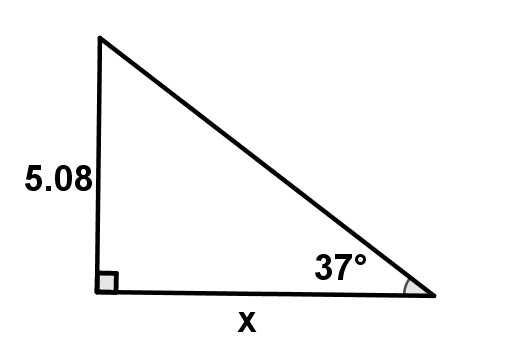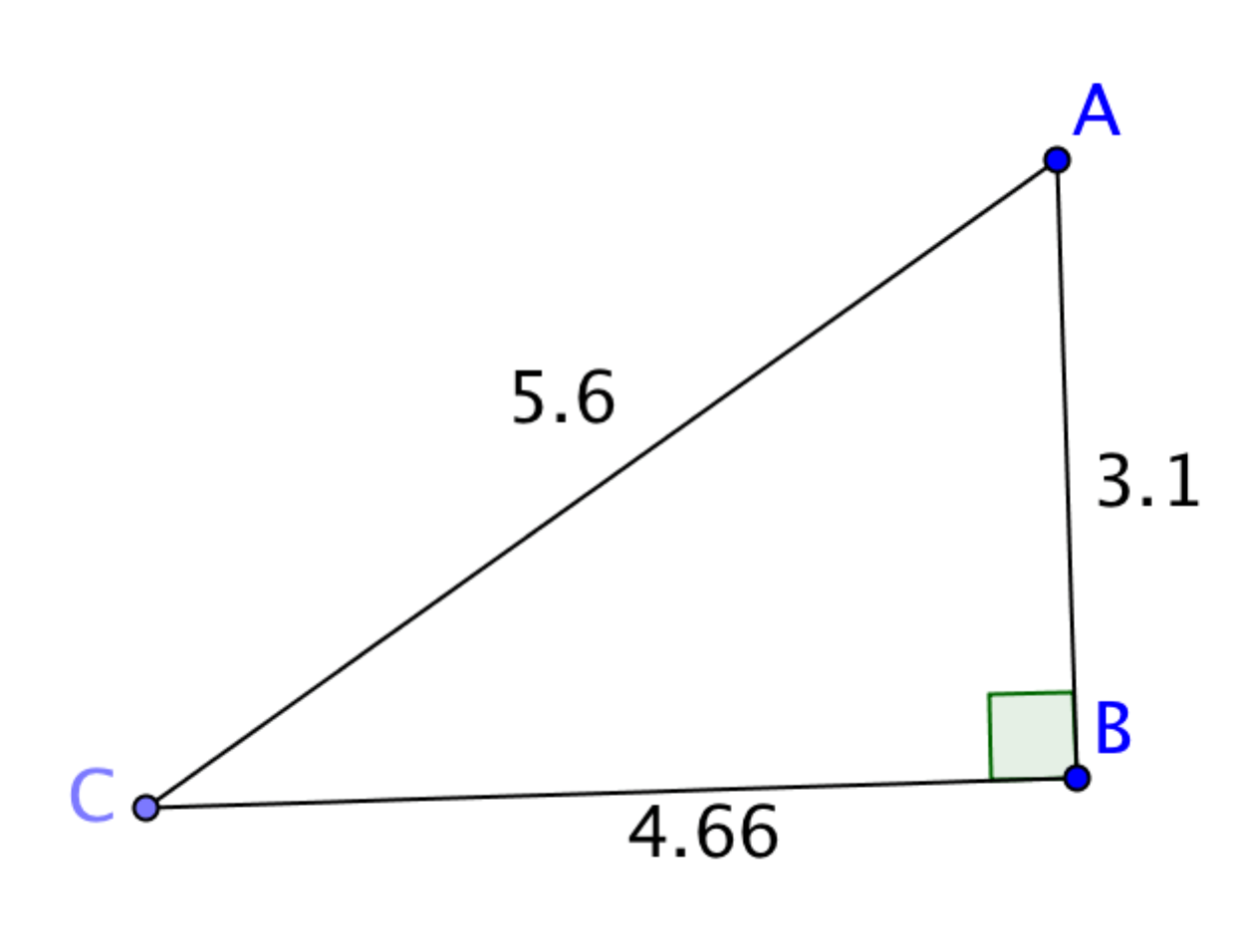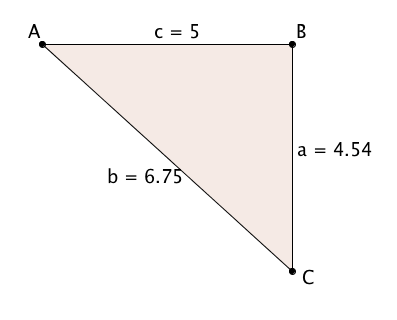# Right Triangles and Trigonometry

## Objective

Find the angle measure given two sides using inverse trigonometric functions.

## Common Core Standards

### Core Standards

?

• G.SRT.C.8 — Use trigonometric ratios and the Pythagorean Theorem to solve right triangles in applied problems. Modeling is best interpreted not as a collection of isolated topics but in relation to other standards. Making mathematical models is a Standard for Mathematical Practice, and specific modeling standards appear throughout the high school standards indicated by a star symbol (★). The star symbol sometimes appears on the heading for a group of standards; in that case, it should be understood to apply to all standards in that group.

## Criteria for Success

?

1. Identify sine, cosine, and tangent as trigonometric functions as well as relationships between side lengths in right triangles.
2. Describe that because they are functions, we need to be able to UNDO them (just like addition, subtraction, etc.).
3. Calculate the inverse of sine, cosine, and tangent using technology—called arcsine, arccosine, and arctangent. They are also noted as ${\mathrm{sin}^{-1}(x)}$, ${\mathrm{cos}^{-1}(x)}$, and ${\mathrm{tan}^{-1}(x)}$ respectively.
4. Use the understanding that when you give the arcsine of a value, you are saying, “What angle gives me a sine of ______?” to solve problems.
5. Use the inverse trigonometric functions to find the angle measures from the given ratios.

## Tips for Teachers

?

• This lesson is an extension of G-SRT.8, which does not specifically mention using inverse trigonometric function. This lesson will prepare students to access F.BF.4 standard in Algebra II.
• Anchor Problem #1 will require the teacher to do a significant amount of modeling to define the inverse of sine, cosine, and tangent as arcsine, arccosine, and arctangent, respectively.

## Anchor Problems

?

### Problem 1

Find the value of the side length marked $x$.### Problem 2

Given the following right triangle, find all of the angle measures to the nearest degree.## Problem Set

?

The following resources include problems and activities aligned to the objective of the lesson that can be used to create your own problem set.

• Include problems where students are given a real-life scenario and they must find the missing angles and side-length measures.
• Include problems where students need to identify at least two ways of solving a problem—either using the Pythagorean theorem, trigonometric ratios, or inverse trigonometric ratios.
• Include error analysis where the error is using the incorrect trigonometric ratio to find a missing value.

Find the measure of ${\angle CAB}$. Round angle measures to the nearest degree. Use two different trigonometric ratios.